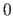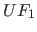Next: Addition Up: Functions Previous: Numbers and variables as   Contents   Index

### Real subfunctions

Every real subfunction, like each variable, represents one value. Before evaluing a subfunction, the values of its subfunctions will be evalued.

There are no volatile / non-defined values. Function values, which are not defined for the normal mathematical function, are mapped to. Since for Fib objects no claim is made that they are mathematically correct (they should be unambiguous, reproducible and evaluable), so the filling of gaps in the mathematically definition is appropriate.

In the followingandare subfunctions.

Subsections

Betti Österholz 2013-02-13